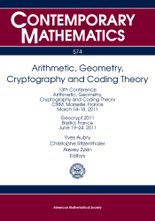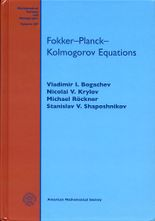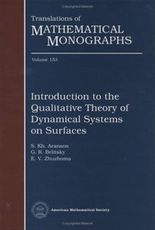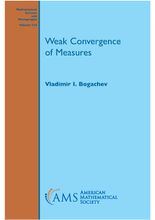• A
• A
• A
• ABC
• ABC
• ABC
• А
• А
• А
• А
• А
Regular version of the site
Of all publications in the section: 13
Sort:
by name
by yearBook
Iss. 574. Providence: American Mathematical Society, 2012.

The volume is to contain the proceedings of the 13th conference AGCT as well as the proceedings of the conference Geocrypt. The conferences focus on various aspects of arithmetic and algebraic geometry, number theory, coding theory and cryptography. The main topics discussed at conferences include the theory of curves over finite fields, theory of abelian varieties both over global and finite fields, theory of zeta-functions and L-functions, asymptotic problems in number theory and algebraic geometry, algorithmic aspects of the theory of curves and abelian varieties, the theory of error-correcting coding and particularly that of algebro-geometric codes, cryptographic issues related to algebraic curves and abelian varieties.Book
Bogachev V. Providence: American Mathematical Society, 2010.

The book gives a systematic account of the theory of differentiable measures and the Malliavin calculus.Book
Bogachev V., Krylov N. V., Roeckner M. et al. Providence: American Mathematical Society, 2015.

This book gives an exposition of the principal concepts and results related to second order elliptic and parabolic equations for measures, the main examples of which are  Fokker-Planck-Kolmogorov equations for stationary and transition probabilities of diffusion  processes. Existence and uniqueness of solutions are studied along with existence  and Sobolev regularity of their densities and upper and lower bounds for the latter. The target readership includes mathematicians and physicists whose research is related to diffusion processes as well as elliptic and parabolic equations.Book
Bogachev V. Providence: American Mathematical Society, 1998.

This book presents a systematic exposition of the modern theory of Gaussian measures. The basic properties of finite and infinite dimensional Gaussian distributions, including their linear and nonlinear transformations, are discussed. The book is intended for graduate students and researchers in probability theory, mathematical statistics, functional analysis, and mathematical physics. It contains a lot of examples and exercises. The bibliography contains 844 items; the detailed bibliographical comments and subject index are included.Book
Edited by: I. M. Krichever, V. M. Buchstaber. American Mathematical Society, 2008.

This volume contains a selection of papers based on presentations given in 2006-2007 at the S. P. Novikov Seminar at the Steklov Mathematical Institute in Moscow. Novikov's diverse interests are reflected in the topics presented in the book. The articles address topics in geometry, topology, and mathematical physics. The volume is suitable for graduate students and researchers interested in the corresponding areas of mathematics and physics.Book
Providence: American Mathematical Society, 2005.

Idempotent mathematics is a rapidly developing new branch of the mathematical sciences that is closely related to mathematical physics. The existing literature on the subject is vast and includes numerous books and journal papers. A workshop was organized at the Erwin Schrodinger Institute for Mathematical Physics (Vienna) to give a snapshot of modern idempotent mathematics. This volume contains articles stemming from that event. Also included is an introductory paper by G. Litvinovand additional invited contributions. The resulting volume presents a comprehensive overview of the state of the art. It is suitable for graduate students and researchers interested in idempotent mathematics and tropical mathematics.Book
V.A.Vassiliev. Providence: American Mathematical Society, 2001.Book
Shen A., Uspensky V. A., Vereshchagin N. American Mathematical Society, 2017.Book
Polishchuk A., Positselski L. Providence: American Mathematical Society, 2005.Book
Vol. 621. American Mathematical Society, 2014.
The purpose of this volume is to both celebrate half a century of Mathematics at the Center for Research and Advanced Studies in Mexico City (Cinvestav) and the opening of the Institute for Geometry and Physics Miami-Cinvestav-Campinas.Book
Edited by: V. Buchstaber, B. Dubrovin, I. M. Krichever. Providence: American Mathematical Society, 2014.Book
Aranson S., Belitsky G., Zhuzhoma E. V. Vol. 153: Introduction to the qualitative theory of dynamical systems on surfaces. American Mathematical Society, 1996.

The book is an introduction to the qualitative theory of dynamical systems on manifolds of low dimension (on the circle and on surfaces). Along with classical results, it reflects the most significant achevements in this area obtained in recent times. The reader of this book need to be familiar only with basic courses in differential equations and smooth manifolds.